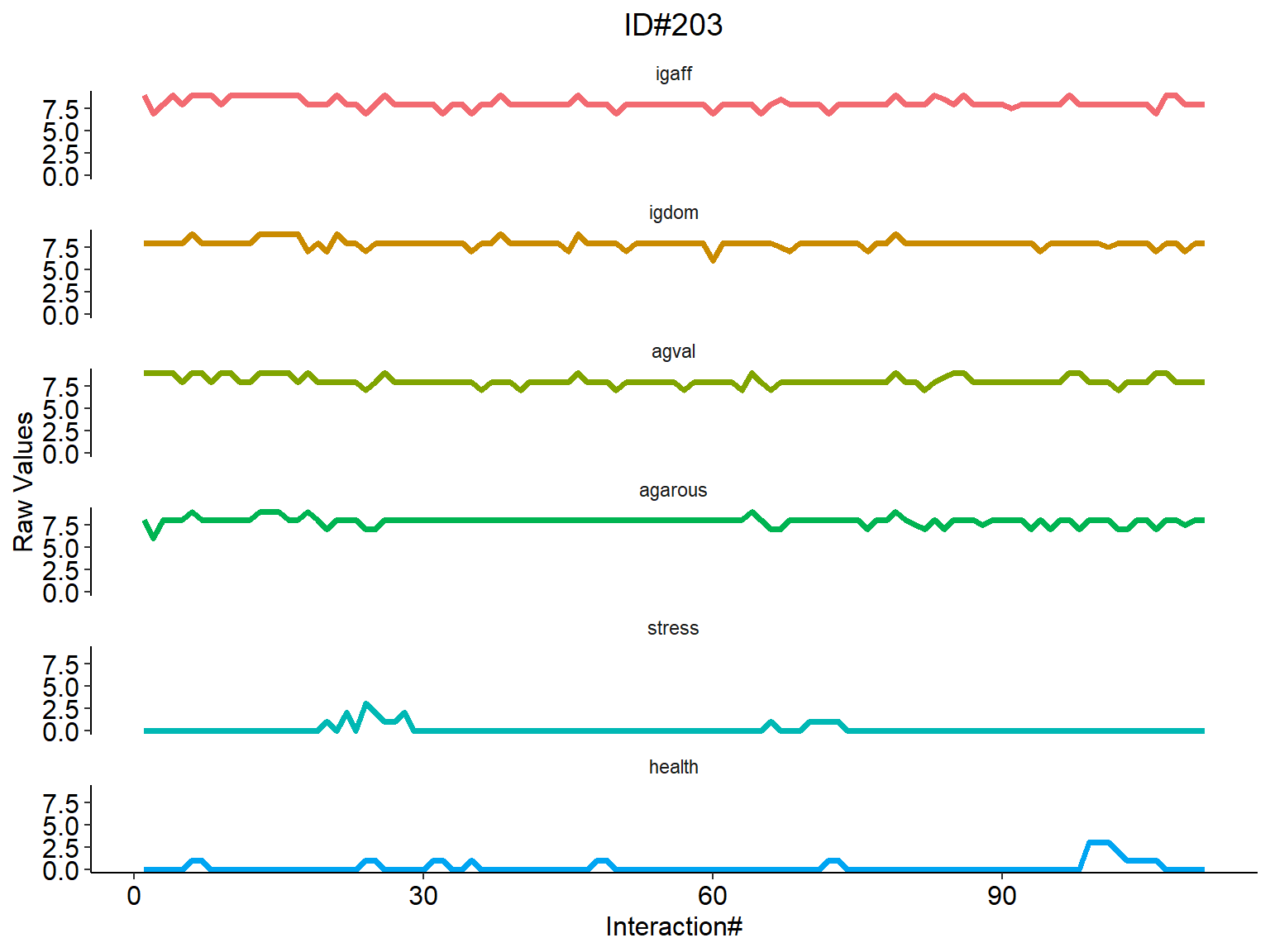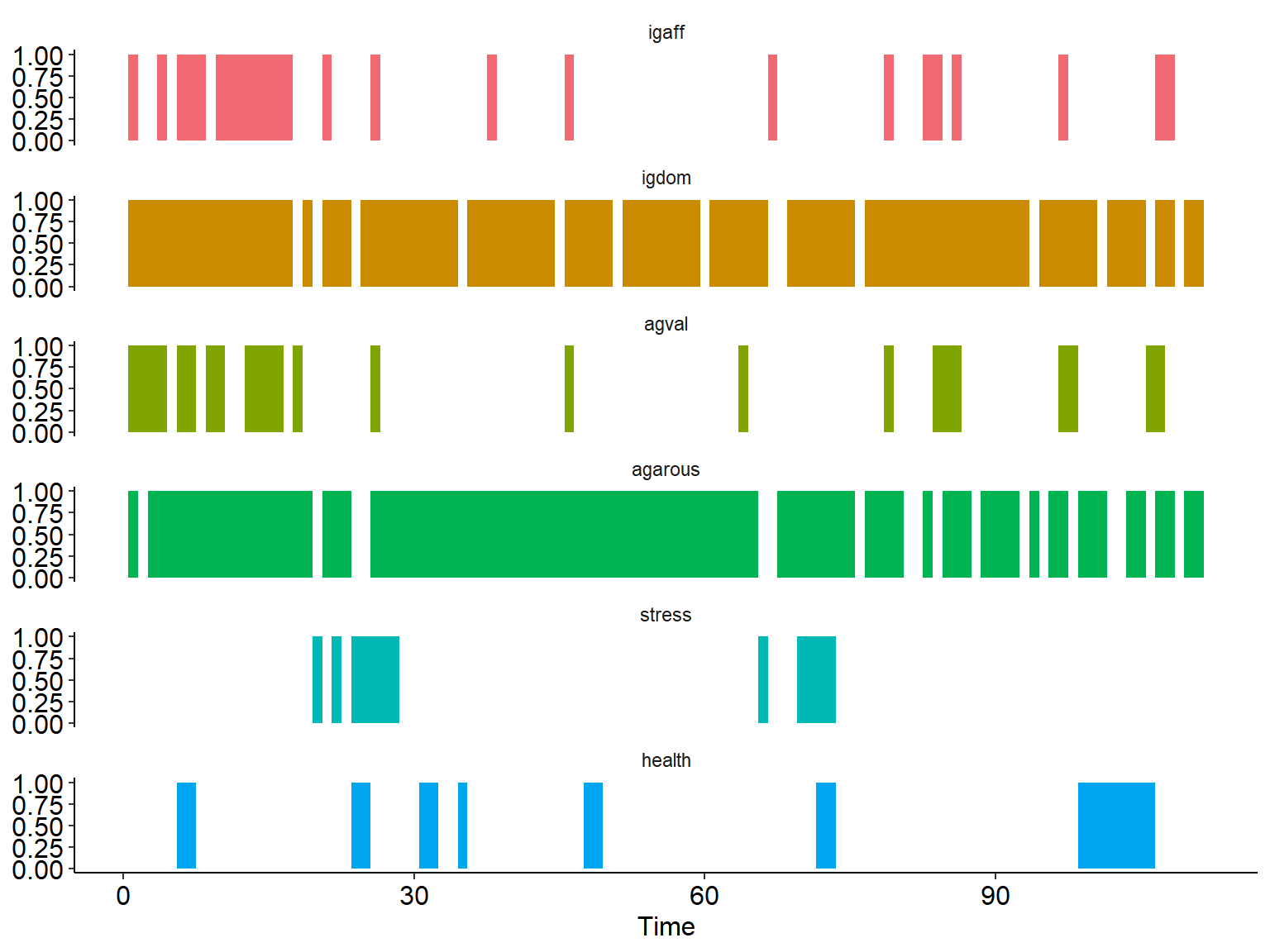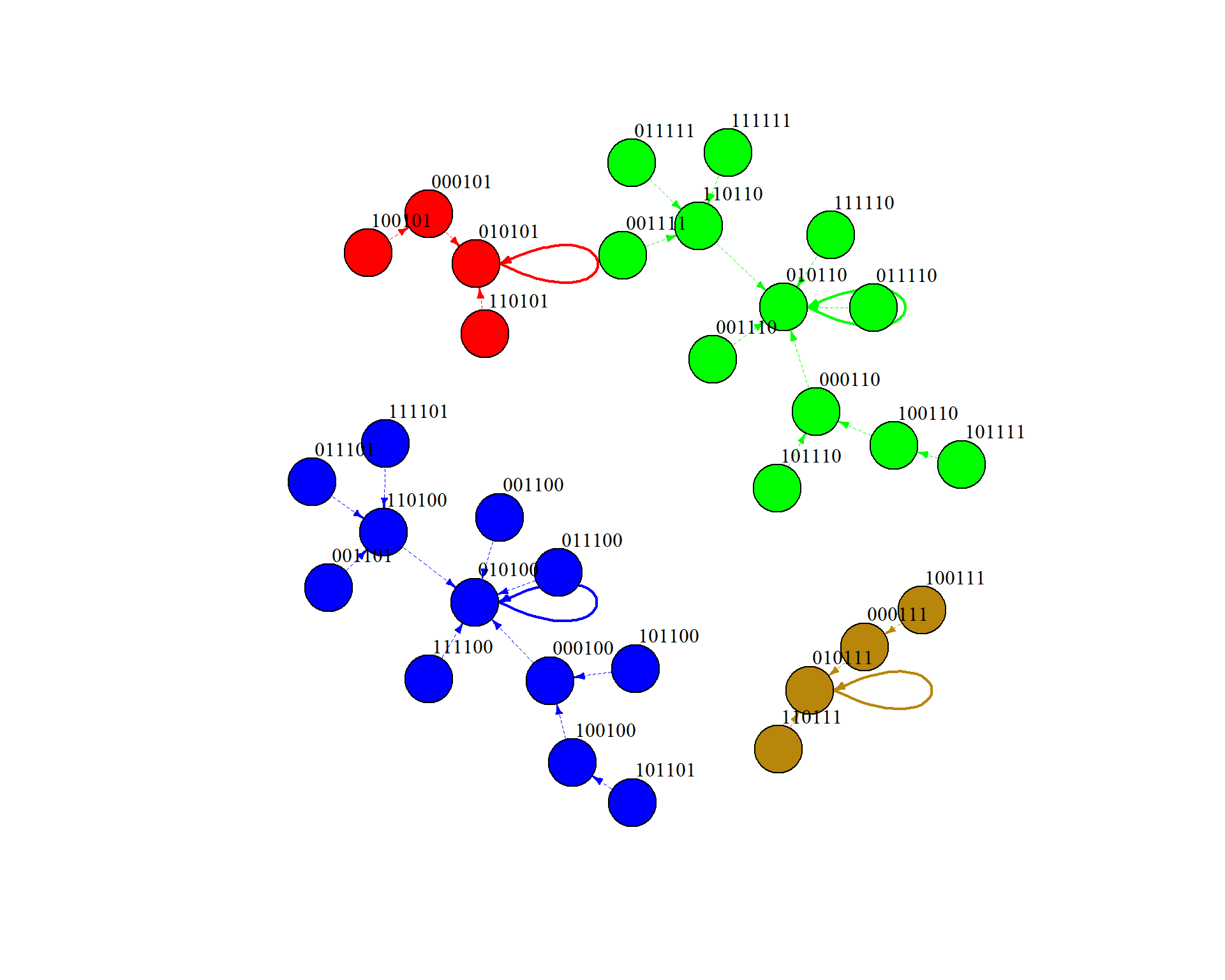## Overview

Dynamical system methods have been instrumental in explaining mechanisms of change and predicting future trajectories and states of psychological systems (Boker & Graham, 1998; Chow, Ferrer, & Hsieh, 2010). A natural next step is to use these methods to design control strategies and guide system trajectories (Molenaar & Nesselroade, 2015; Wang et al., 2014).

In this tutorial, we introduce and forward a Boolean network method because it can give an intuitive explanation of psychological dynamics that are useful for theorists and suggestions for network control that are useful for practitioners. We demonstrate the utility of the Boolean network through analysis of multivariate binary time-series data simulated to mimic intensive longitudinal data (e.g., ecological momentary assessment).

## Boolean network

Boolean network (Kauffman, 1969, 1993) method is a specific instantiation of discrete dynamical system model. The special characteristic of the Boolean network method is to use the Boolean operators, including AND (&), OR (|), or NOT (!), to describe and model temporal dynamics between variables.

The Boolean network method is like a vector-autoregression (VAR) model, whereas the temporal relations are expressed using the Boolean operators, and the variables are binary.

Data type that can use the Boolean network method: TThe Boolean netowrk method can be applied on intensive longitudinal data (e.g., ecological Momentary Assessment, physiological data, physical activity, behavior codes) to model the temporal dynamics and facilitate control design. The method can work on continuous-scale time-series, which need to be binarized and we show it in this tutorial; it can also work on binary time-series data, which I show in the “Tutorial of Boolean Network Analysis of Time-Series Data - Part 1 Binary Data”.

We are using the R package BoolNet (Mussel et al., 2010; Lähdesmäki et al., 2003) to conduct the data analysis in this tutorial.

This tutorial is to demonstrate the following 6 steps of Boolean network approach, including:

1. Read in continuous time data

2. Binarization of continuous data

3. Inference of Boolean functions

4. Plotting state space transition graph

5. Extraction of attractors

6. Network control (under development)

## Step 1: Read in continuous-scale time-series data

### What dataset is used in this tutorial?

Here we use the AMIB data collected by Dr. Nilam Ram and his colleauges. AMIB is an experience sampling dataset, or sometimes called intensive longitudinal data. Each person reports theirs emotions and behaviors multiple times a day and mulitple days.

Note: If you have intensive longitudinal data on a continuous scale, then you can go to step 2 to binarize your data.

We make use of the extended AMIB data. Specifically, the Phase 2 interaction-level data. This group of 30 participants provided up to 8 reports per day for 21 days. Thus, the time-series are relatively long (> 100 observations per person, M = 140, range = 64-168).

#set filepath for data file
filepath <- "https://quantdev.ssri.psu.edu/sites/qdev/files/AMIBshare_phase2_interaction_2019_0501.csv"
#read in the .csv file using the url() function
AMIB_interactionP2 <- read.csv(file=url(filepath),header=TRUE)

For the purpose of illustrating the Boolean network method we use a not-small (to be truly multivariate) and not-large (for pedagogy) subset of variables:

Person index: “id”

Time indices: “day” (1-21) and

“interaction” (1-168)

Six variables of interest: “igaff”, “igdom”, “agval”, “agarous”“,”stress“, and”health" (interpersonal affection, interpersonal dominance, affect valence, affect arousal, stress, health[reverse coded])

Subsetting data to variables of interest.

#subsetting to vaiables of interest
AMIB_interactionP2 <- AMIB_interactionP2[ ,c("id","day","interaction",
"igaff","igdom",
"agval", "agarous",
"stress", "health")]
describe(AMIB_interactionP2)

Note that the variables are on different scales (e.g., 1-9, 0-5).

The Boolean network model is a person-specific model, meaning that each person is modeled separately - one model for a person. We illustrate fitting for one person, and then indicate how we loop through many persons to obtain models for each person. Subsetting to one person’s data (id#203)

#select one person's data
data_indiv <- AMIB_interactionP2[AMIB_interactionP2$id == 203, ] describe(data_indiv) #### Plot the simulated continuous time-series # specify the colors of the time-series n = 6 cols = gg_color_hue(n) data_indiv.melt <- melt(data_indiv, id = c("id","day","interaction")) #plotting intraindividual change ggplot(data = data_indiv.melt, aes(x = interaction, y = value, group= variable, color = variable))+ geom_line(size = 1.4) + scale_color_manual(values = cols) + facet_wrap(~variable, ncol = 1) + #plot layouts scale_x_continuous(name="Interaction#") + scale_y_continuous(name="Raw Values") + # theme_classic() + theme( strip.background = element_blank(), panel.background = element_blank(), legend.title = element_blank(), legend.key = element_blank(), legend.position = "none", axis.text.y=element_text(color="black",size=12), axis.text.x=element_text(color="black",size=12), axis.title.y=element_text(color="black",size=12), axis.title.x=element_text(color="black",size=12), axis.line = element_line(color = 'black'), plot.title=element_text(size=14, hjust=.5)) + ggtitle("ID#203")Here it is easy to note that the variables are on different scales (e.g., 1-9, 0-5). ## Step 2: Binarization of continuous data Binarization is to convert the continuous-scale variables to binary variables, so that it can be fitted into the Boolean model. This is done using the “binarizeTimeSeries” function in BoolNet package, and note here the binarization is done by rows, instead of by columns. Since we usually use the long-format to organize intensive longitudinal data, we take the transpose here to transform the data into a format that each row is a variable. The “binarizeTimeSeries” function is using k-means clustering and k (number of groups) = 2. data_indiv$id <-NULL # remove the id index
data_indiv$day <-NULL # remove the id index data_indiv$interaction <-NULL # remove the id index
data_indiv <- t(data_indiv)

# binarize the continuous-scale variables
bin.time.series <- binarizeTimeSeries(data_indiv)

# print the first five time points
print(bin.time.series$binarizedMeasurements[,1:5]) ## 1 2 3 4 5 ## igaff 1 0 0 1 0 ## igdom 1 1 1 1 1 ## agval 1 1 1 1 0 ## agarous 1 0 1 1 1 ## stress 0 0 0 0 0 ## health 0 0 0 0 0 bin.time.series$thresholds
##     igaff     igdom     agval   agarous    stress    health
## 8.4247674 7.5515464 8.4378592 7.5515233 0.7083333 0.6842105

We can check the threshold of each variable. binarizeTimeSeries use K-means to split the variable by the threshold. E.g., any igaff value above 8.4247674 will be converted to 1, otherwise 0.

#### Plot the binary time-series

Here we plot the converted time-series, and now it is in the binary format. Any color indicates the variable is 1, and white space indicates the variable is 0.

# for plotting purposes, convert the binary time-series back to long-format
bin.data.plot <- bin.time.series$binarizedMeasurements bin.data.plot <- t(bin.data.plot) bin.data.plot <- data.frame(bin.data.plot) bin.data.plot$index <- 1:nrow(bin.data.plot)

bin.data.plot.melt <- melt(bin.data.plot,id = "index")

bin.data.plot.melt$value.variable <- ifelse(bin.data.plot.melt$value==0,"0",                                        as.character(bin.data.plot.melt$variable)) bin.data.plot.melt$value.variable <- factor(bin.data.plot.melt$value.variable, levels = c("0","igaff","igdom", "agval","agarous","stress","health")) # specify the colors of the time-series n = 6 cols = gg_color_hue(n) ggplot(data = bin.data.plot.melt)+ geom_rect(aes(xmin = index - .5, xmax = index + .5, ymin = 0, ymax = 1, fill = factor( value.variable)))+ facet_wrap(~variable, ncol = 1) + scale_fill_manual(values = c("#FFFFFF", cols))+ theme( strip.background = element_blank(), panel.background = element_blank(), legend.title = element_blank(), legend.key = element_blank(), legend.position = "none", axis.text.y=element_text(color="black",size=12), axis.text.x=element_text(color="black",size=12), axis.title.y=element_text(color="black",size=12), axis.title.x=element_text(color="black",size=12), axis.line = element_line(color = 'black'))+ ylim(0,1)+ xlab("Time")Now you can compare the continuous scale plot and the binary scale plot, to see how the values are converted. ## Step 3: Inference of Boolean functions net.data <- bin.time.series$binarizedMeasurements
network.size<- 6

booleannet <- reconstructNetwork(net.data,
method = "bestfit",
maxK = 2,
returnPBN = F)
print(booleannet)
## Probabilistic Boolean network with 6 genes
##
## Involved genes:
## igaff igdom agval agarous stress health
##
## Transition functions:
##
## Alternative transition functions for gene igaff:
## igaff = (agval & health) (error: 21)
##
## Alternative transition functions for gene igdom:
## igdom = (!igaff) | (igdom) (error: 13)
##
## Alternative transition functions for gene agval:
## agval = (agval & !agarous) (error: 21)
## agval = (!igaff & agval) (error: 21)
##
## Alternative transition functions for gene agarous:
## agarous = 1 (error: 18)
##
## Alternative transition functions for gene stress:
## stress = (stress) (error: 10)
##
## Alternative transition functions for gene health:
## health = (!agval & health) (error: 13)
##
## Knocked-out and over-expressed genes:
## agarous = 1
# saveNetwork(net, paste(outputpath, id,"-network.txt", sep = "")) # can't save a collection of BN

### How Boolean functions are inferred?

The details of how the Boolean functions are inferred can be found in Akutsu et al. (2000). The essence is to compare the outcome varible’s time-series at time t+1 and the time-series of the input variables at time t, after appyling the Boolean operator AND, OR, or NOT.

### How to interpret the Boolean functions?

Take function igaff = (agval & health) as an example:

This indicates igaff(t+1) is predicted by agval(t) AND health(t), which means interpersonal affect (igaff) is only 1 when both affect valence (agval) and health are both 1. This partipant can only feel interpersonal affection when he/she was feeling postive affect himeself/herself, and was not bothered by low health.

### What is error rate?

Error rate here is the sum of false negative (predicting the outcome as 0 when it was actually 1) and false positive (predicting the outcome as 1 when it was actually 0). Note the total number of time points is 6 in the simulated time-series, and error rate = 21 indicates out of 6 time points 21 were predicted incorrectly using the “bestfit” algorithm.

Here, we select the fisrt network if more than 1 rule inferred per node, so that the attractors can be extracted.

# note here we only chose the first function of all possible functions
singlenet <- chooseNetwork(booleannet,
functionIndices = rep(1,network.size),
dontCareValues=rep(1,network.size),
print(singlenet)
## Boolean network with 6 genes
##
## Involved genes:
## igaff igdom agval agarous stress health
##
## Transition functions:
## igaff = (agval & health)
## igdom = (!igaff) | (igdom)
## agval = (agval & !agarous)
## agarous = 1
## stress = (stress)
## health = (!agval & health)
##
## Knocked-out and over-expressed genes:
## agarous = 1

### Why extract attractors first?

Note here, we extract attractors first (Step 5) because state transition graph needs the attractor object as an input. But we will explain what the attractors mean in Step 3, as the state transition graph (Step 4) will help you understand the concept of attractor.

ga <- getAttractors(network = singlenet,
returnTable = TRUE)

# print(ga, activeOnly=TRUE)

# Step 4: Plot state space transition graph

p<-plotStateGraph(ga,
piecewise=TRUE,
drawLabels = T,
plotIt = F,
colorsAlpha = c(colorBasinsNodeAlpha    = 1,
colorBasinsEdgeAlpha    = 1,
colorAttractorNodeAlpha = 1,
colorAttractorEdgeAlpha = 1))
plot.igraph(p,
label.cex = 1.2,
vertex.label.color="black",
vertex.label.dist=2,
remove.loops = T,
edge.arrow.size=.4)### What does state transition graph mean?

Plot the state transition graph, where each node is a state of the system (a vector of state of the six variables), and the arrows/edges are state-to-state transition. E.g., state 100101 means (igaff = 1, igdom = 0, agval = 0, agarou = 1, stress = 0, health = 1); and the state 100101 will transition to state 000101 (marked as red). The state transition is computed based on the Boolean functions inferred in the first step. e.g., because the igaff(t+1) = agval(t) & health(t), so when agval(t) = 0 and health(t) = 1, igaff(t+1) = 0 & 1 = 0, Similarly, the other 5 variables can be computed, so the next state of the system is (0,0,0,1,0,1), hence state 100101 transitions to state 000101.

### What is an attractor and how can I tell from the state transition graph?

When a state transitions back to itself, it is an attractor - as the system gravitates toward an attractor. Here in each attractor, there is 1 or multiple attractor state(s) that the system tends to stay for a long run.

In the state transition graph, the attractor can be found by the self-loop, indicating the state transitions back to itself, e.g., state 010101 (marked in red) has a self-loop, and it is the attractor state.

### What are the possible applications in psychology?

In psychology, attractors can have different desirability based on practical concerns. For example, an attractor that has stress = 1 is considered undesirable, e.g., 010110 (marked in green) where the second last digit indicates stress = 1 in this attractor. In comparison, an attractor that has stress = 0 is more desirable, e.g., 010101 (marked in red).

## Step 5: Extraction of attractors

# ga <- getAttractors(network = singlenet,
#                   returnTable = TRUE)

print(ga, activeOnly=TRUE)
## Attractor 1 is a simple attractor consisting of 1 state(s) and has a basin of 12 state(s).
## Active genes in the attractor state(s):
## State 1: igdom, agarous
##
## Attractor 2 is a simple attractor consisting of 1 state(s) and has a basin of 12 state(s).
## Active genes in the attractor state(s):
## State 1: igdom, agarous, stress
##
## Attractor 3 is a simple attractor consisting of 1 state(s) and has a basin of 4 state(s).
## Active genes in the attractor state(s):
## State 1: igdom, agarous, health
##
## Attractor 4 is a simple attractor consisting of 1 state(s) and has a basin of 4 state(s).
## Active genes in the attractor state(s):
## State 1: igdom, agarous, stress, health

### What do we know about the attractors?

We already extracted attractors and explained them previously, here we print out all the attractors and which nodes are activated in each attractor state.

There are 4 attractors found in this system, and the active nodes in the attractor state are shown repsectively. E.g., attractor 1 (marked by blue) have all 2 nodes turned ON in the attractor state, interpersonal dominance (igdom) and affect arousal (agarous).

## Step 6: Network control (under development)

### Why network control is useful?

After extracting the attractors, we can define its desirability based on practical concerns. Using the parent-child dynamic example, we might want to direct the parent and/or child out of a yelling attractor. That is why network control is useful.

### How to design network control?

Network control can be designed based on a search algorithm to find the node to flip so that the system can move from the undesirable attractors to the desirable attractor, as an intervention.

### What are the possible applications in psychology?

For a psychological sytem, there could be a node flip to direct the system out of an undesirable state to a desirable state, e.g., an attractor with stress = 1 to another attractor stress = 0. This method can be applied in personalized intervention, to prevent indivduals from being stuck in undesirable states.

### To be continued

This step is currently under development and testing, so come back after a month or so, when I finish the development and testing of the algorithm!